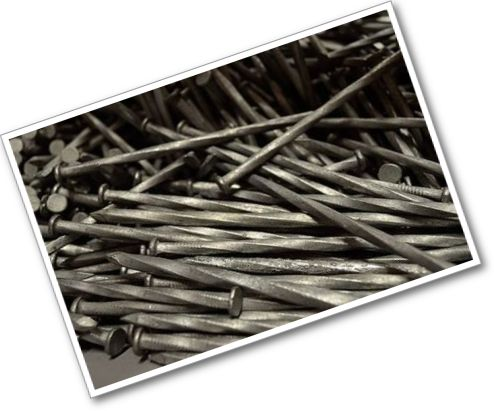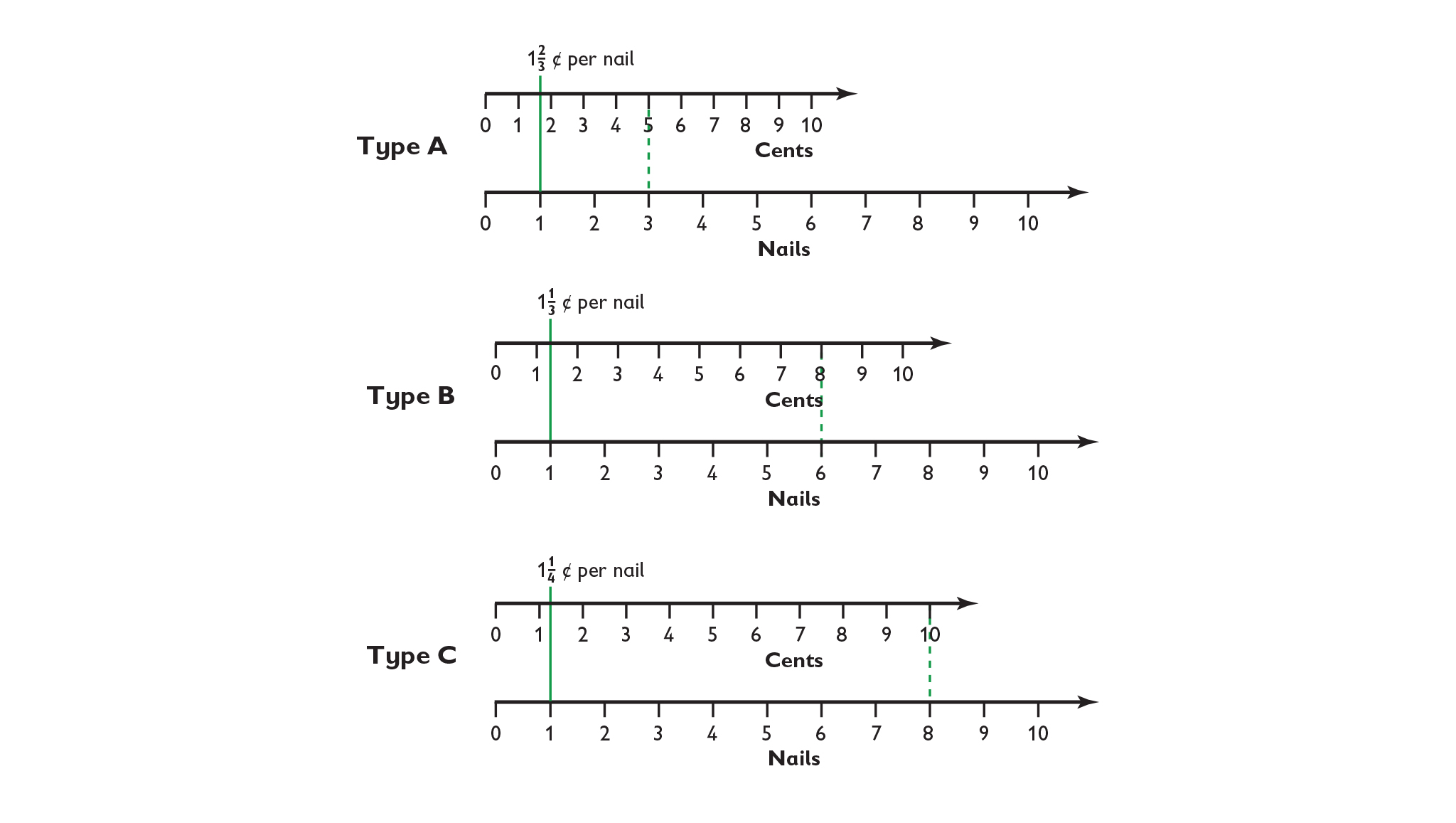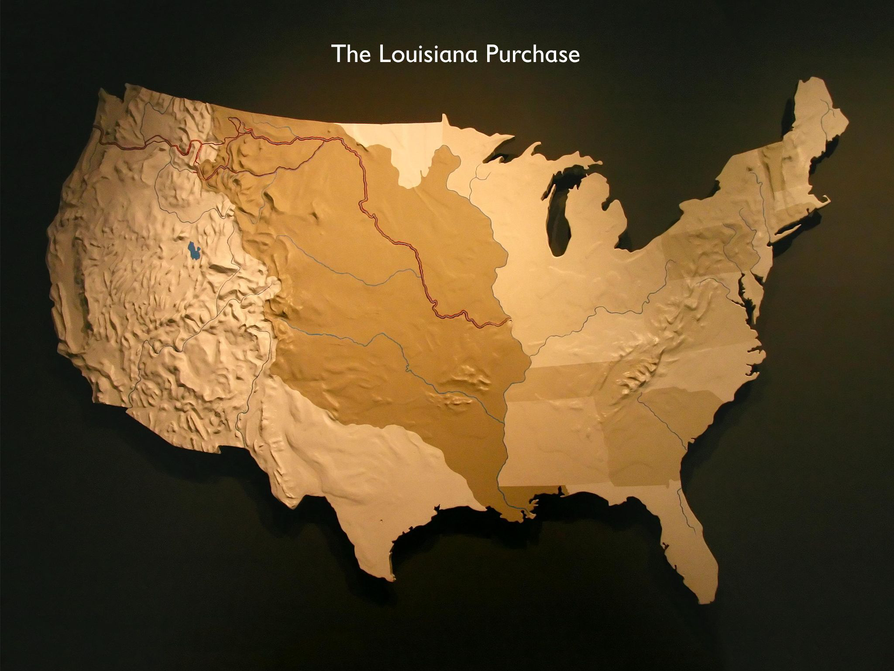Subject:
Mathematics
Material Type:
Lesson Plan
Level:
Middle School
7
Provider:
Pearson
Tags:
• Problem Solving
Language:
English
Media Formats:

# Gallery Problems Exercise## Overview

Allow students who have a clear understanding of the content thus far in the unit to work on Gallery problems of their choosing. You can then use this time to provide additional help to students who need review of the unit's concepts or to assist students who may have fallen behind on work.

# Gallery Overview

Represent a Math Problem

Explore ways to represent math problems—make an equation using a double number line, a ratio table, and a tape diagram. Then solve a problem comparing the prices of different types of nails using one of the representations.

Research Ratios and Rates

Research how ratios and rates are related to proportional relationships. Watch the video tutorials and explore the other resources.

Louisiana Purchase

Solve a problem about the Louisiana Purchase.

# Represent a Math Problem

1. Answers will vary. Students should explain what they learned when exploring the representation.

2. The cost-per-nail rate for each type of nail is written below:Type A:
Type B:
Type C:
Type C costs the least cents per nail.

3. Answers will vary. Possible answer: Jack did not pay attention to the units in the number. Jack wrote the quotient of nails per cent, but made a mistake by thinking the least nails per cent as the cheapest. It is actually the most expensive. Using his methods, he could find the cheapest by looking at which one has the most nails per cent. It would be useful to make the rates comparable by converting the fractions to the same denominator or using decimals.

# Represent a Math Problem

Explore each of these math representations: a ratio table tool, a double number line tool, and a tape diagram tool.

1. Research each type of representation and explore how to use it. Select one representation to describe to the class.
2. Solve this problem. Represent it using a double number line or a ratio table.

Three types of nails are for sale:

• It costs 5¢ to buy 3 type A nails.
• It costs 8¢ to buy 6 type B nails.
• It costs 10¢ to buy 8 type C nails.

Which type of nails costs the least per nail?

1. Jack says:

“The rates are $\frac{3}{5}$ for type A, $\frac{6}{8}$ for type B, and $\frac{8}{10}$ for type C. Type A costs the least per nail." Jack is wrong. Explain his mistake.

# Research Ratios and Rates

Do some research to learn about how ratios and rates are related to proportional relationships.

• Search using a few different keywords to find resources. Follow any links that look useful.
• Watch video tutorials about proportional relationships.
• Review worked examples of problems using proportional relationships.
• Look up ratios and rates in the dictionary.

1. How are ratios and rates related to proportional relationships?
2. What are five things you think you will learn about proportional relationships this year?
3. When is it useful to research a concept?

# Louisiana Purchase

1. Acres of land west of the Mississippi

$\begin{array}{c}800,000\text{sq mi}•640\frac{\text{\hspace{0.17em}}\text{acres}}{\text{sq mi}}=512,000,000\text{\hspace{0.17em}}\text{acres}\\ \frac{\text{cost}}{\text{\hspace{0.17em}}\text{acre}}=\frac{\text{}15,000,000}{\text{512,000,000}}\\ \frac{\text{}15}{512}\approx \text{}0.0293\text{per acre}\\ \text{}0.0293=2.93\text{cents per acre}\end{array}$

The 800,000 sq mi of land west of the Mississippi River was purchased from France for about 3 cents per acre in 1803.

2. Estimates will vary. Possible estimate: The acres in the land west of the Mississippi River can be overestimated as 800,000,000 acres. I can easily find $16 million divided by 800 million. The price should be around$0.02 or 2 cents per acre.

3. Diagrams will vary. Check students' work.

# Louisiana Purchase

• Solve this problem.

In 1803, the United States negotiated the Louisiana Purchase with France and purchased approximately 800,000 sq mi of land west of the Mississippi River for \$15 million.

Reminder: An acre is a measure of area. There are 640 acres in a square mile.

1. How much did the land cost per acre?
2. Make an estimate.
3. Create a diagram to represent the situation.